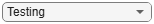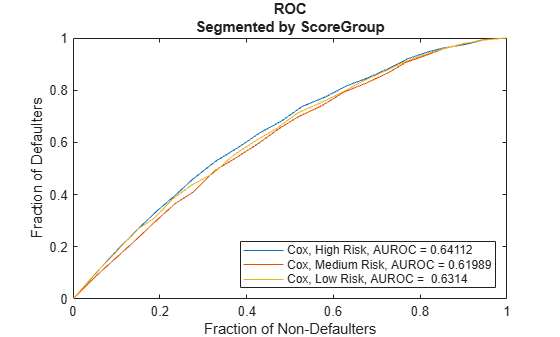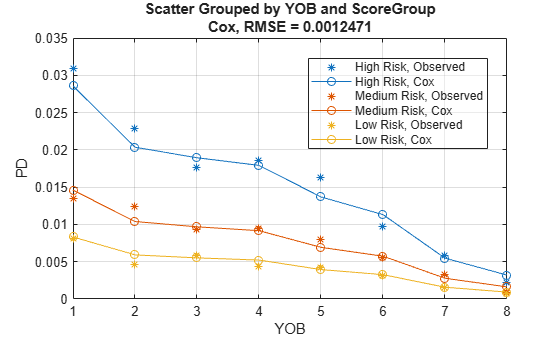# Cox

Create `Cox` model object for lifetime probability of default

## Description

Create and analyze a `Cox` model object to calculate lifetime probability of default (PD) using this workflow:

1. Use `fitLifetimePDModel` to create a `Cox` model object.

2. Use `predict` to predict the conditional PD and `predictLifetime` to predict the lifetime PD.

3. Use `modelDiscrimination` to return AUROC and ROC data. You can plot the results using `modelDiscriminationPlot`.

4. Use `modelAccuracy` to return the root mean square error (RMSE) of observed and predicted PD data. You can plot the results using `modelAccuracyPlot`.

## Creation

### Syntax

``CoxPDModel = fitLifetimePDModel(data,ModelType,AgeVar=agevar_value)``
``CoxPDModel = fitLifetimePDModel(___,Name=Value)``

### Description

example

````CoxPDModel = fitLifetimePDModel(data,ModelType,AgeVar=agevar_value)` creates a `Cox` PD model object. If you do not specify variable information for `IDVar`, `LoanVars`, `MacroVars`, and `ResponseVar`, then: `IDVar` is set to the first column in the `data` input.`LoanVars` is set to include all columns from the second to the second-to-last columns of the `data` input.`ResponseVar` is set to the last column in the `data` input. ```

example

````CoxPDModel = fitLifetimePDModel(___,Name=Value)` sets optional properties using additional name-value arguments in addition to the required arguments in the previous syntax. For example, ```CoxPDModel = fitLifetimePDModel(data(TrainDataInd,:),"Cox",ModelID="Cox_A",Descripion="Cox_model",AgeVar="YOB",IDVar="ID",LoanVars="ScoreGroup",MacroVars={'GDP','Market'},ResponseVar="Default",TimeInterval=1)``` creates a `CoxPDModel` using a `Cox` model type. You can specify multiple name-value arguments. ```

### Input Arguments

expand all

Data, specified as a table, in panel data form. The data must contain an `ID` column and an `Age` column. The response variable must be a binary variable with the value `0` or `1`, with `1` indicating default.

Data Types: `table`

Model type, specified as a string with the value `"Cox"` or a character vector with the value `'Cox'`.

Data Types: `char` | `string`

Name-Value Arguments

Specify required and optional pairs of arguments as `Name1=Value1,...,NameN=ValueN`, where `Name` is the argument name and `Value` is the corresponding value. Name-value arguments must appear after other arguments, but the order of the pairs does not matter.

Example: ```CoxPDModel = fitLifetimePDModel(data(TrainDataInd,:),"Cox",ModelID="Cox_A",Descripion="Cox_model",AgeVar="YOB",IDVar="ID",LoanVars="ScoreGroup",MacroVars={'GDP','Market'},ResponseVar="Default",TimeInterval=1)```

Required `Cox` Name-Value Argument

expand all

Age variable indicating which column in `data` contains the loan age information, specified as `AgeVar` and a string or character vector.

Note

The required name-value argument `AgeVar` is not treated as a predictor in the `Cox` lifetime PD model. When using a `Cox` model, you must specify predictor variables using `LoanVars` or `MacroVars`. The `AgeVar` values are the event times for the underlying Cox proportional hazards model.

`AgeVar` values for each ID should be increasing. If there are nonpositive age increments, `fitLifetimePDModel` warns when you create a `Cox` model and removes the IDs with nonpositive age increments. By default, the `TimeInterval` value is set to the most common age increment in the training data.

Data Types: `string` | `char`

Optional `Cox` Name-Value Arguments

expand all

User-defined model ID, specified as `ModelID` and a string or character vector. The software uses the `ModelID` to format outputs and is expected to be short.

Data Types: `string` | `char`

User-defined description for model, specified as `Description` and a string or character vector.

Data Types: `string` | `char`

ID variable indicating which column in `data` contains the loan or borrower ID, specified as `IDVar` and a string or character vector.

Data Types: `string` | `char`

Loan variables indicating which column in `data` contains the loan-specific information, such as origination score or loan-to-value ratio, specified as `LoanVars` and a string array or cell array of character vectors.

Data Types: `string` | `cell`

Macro variables indicating which column in `data` contains the macroeconomic information, such as gross domestic product (GDP) growth or unemployment rate, specified as `MacroVars` and a string array or cell array of character vectors.

Data Types: `string` | `cell`

Variable indicating which column in `data` contains the response variable, specified as `ResponseVar` and a logical value.

Note

The response variable in the `data` must be a binary variable with `0` or `1` values, with `1` indicating default.

In Cox lifetime PD models, the `ResponseVar` values are define the censoring information for the underlying Cox proportional hazards model.

Data Types: `logical`

Distance between age values in training data in the panel `data` input, specified as `TimeInterval` and a positive numeric scalar.

Use the `TimeInterval` name-value argument to fit time-dependent models and also as the time interval for the PD computation when you use the `predict` function. For example, if the age data (`AgeVar`) is 1, 2, 3, ..., then the `TimeInterval` is `1`; if the age data is 0.25, 0.5, 0.75,..., then the `TimeInterval` is `0.25`. For more information, see Time Interval for Cox Models and Lifetime Prediction and Time Interval.

Note

Unlike `Logistic` and `Probit` models, a `Cox` model requires an `AgeVar` variable. By default, if you do not specify a `TimeInterval` when creating a `Cox` model, the `TimeInterval` is inferred from the increments in the `AgeVar` values in the training `data`.

Data Types: `double`

## Properties

expand all

User-defined model ID, returned as a string.

Data Types: `string`

User-defined description, returned as a string.

Data Types: `string`

Underlying statistical model, returned as a returned as a Cox proportional hazards model object. For more information, see `fitcox` and `CoxModel`.

Data Types: `CoxModel`

ID variable indicating which column in `data` contains the loan or borrower ID, returned as a string.

Data Types: `string`

Age variable indicating which column in `data` contains the loan age information, returned as a string.

Data Types: `string`

Loan variables indicating which column in `data` contains the loan-specific information, returned as a string array.

Data Types: `string`

Macro variables indicating which column in `data` contains the macroeconomic information, returned as a string array.

Data Types: `string`

Variable indicating which column in `data` contains the response variable, returned as a string.

Data Types: `string`

Distance between age values in panel `data` input, returned as a scalar positive numeric.

Data Types: `double`

Extrapolation factor, returned as a positive numeric scalar between `0` and `1`.

By default, the `ExtrapolationFactor` is set to `1`. For age values (`AgeVar`) greater than the maximum age observed in the training data, the conditional PD, computed with `predict`, uses the maximum age observed in the training data. In particular, the predicted PD value is constant if the predictor values do not change and only the age values change when the `ExtrapolationFactor` is `1`. For more information, see Extrapolation for Cox Models, Extrapolation Factor for Cox Models, and Use Cox Lifetime PD Model to Predict Conditional PD.

Data Types: `double`

## Object Functions

 `predict` Compute conditional PD `predictLifetime` Compute cumulative lifetime PD, marginal PD, and survival probability `modelDiscrimination` Compute AUROC and ROC data `modelAccuracy` Compute RMSE of predicted and observed PDs on grouped data `modelDiscriminationPlot` Plot ROC curve `modelAccuracyPlot` Plot observed default rates compared to predicted PDs on grouped data

## Examples

collapse all

This example shows how to use `fitLifetimePDModel` to create a `Cox` model using credit and macroeconomic data.

```load RetailCreditPanelData.mat disp(head(data))```
``` ID ScoreGroup YOB Default Year __ __________ ___ _______ ____ 1 Low Risk 1 0 1997 1 Low Risk 2 0 1998 1 Low Risk 3 0 1999 1 Low Risk 4 0 2000 1 Low Risk 5 0 2001 1 Low Risk 6 0 2002 1 Low Risk 7 0 2003 1 Low Risk 8 0 2004 ```
`disp(head(dataMacro))`
``` Year GDP Market ____ _____ ______ 1997 2.72 7.61 1998 3.57 26.24 1999 2.86 18.1 2000 2.43 3.19 2001 1.26 -10.51 2002 -0.59 -22.95 2003 0.63 2.78 2004 1.85 9.48 ```

Join the two data components into a single data set.

```data = join(data,dataMacro); disp(head(data))```
``` ID ScoreGroup YOB Default Year GDP Market __ __________ ___ _______ ____ _____ ______ 1 Low Risk 1 0 1997 2.72 7.61 1 Low Risk 2 0 1998 3.57 26.24 1 Low Risk 3 0 1999 2.86 18.1 1 Low Risk 4 0 2000 2.43 3.19 1 Low Risk 5 0 2001 1.26 -10.51 1 Low Risk 6 0 2002 -0.59 -22.95 1 Low Risk 7 0 2003 0.63 2.78 1 Low Risk 8 0 2004 1.85 9.48 ```

Partition Data

Separate the data into training and test partitions.

```nIDs = max(data.ID); uniqueIDs = unique(data.ID); rng('default'); % For reproducibility c = cvpartition(nIDs,'HoldOut',0.4); TrainIDInd = training(c); TestIDInd = test(c); TrainDataInd = ismember(data.ID,uniqueIDs(TrainIDInd)); TestDataInd = ismember(data.ID,uniqueIDs(TestIDInd));```

Create a `Cox` Lifetime PD Model

Use `fitLifetimePDModel` to create a `Cox` model using the training data.

```pdModel = fitLifetimePDModel(data(TrainDataInd,:),"Cox",... AgeVar="YOB", ... IDVar="ID", ... LoanVars="ScoreGroup", ... MacroVars={'GDP','Market'}, ... ResponseVar="Default"); disp(pdModel)```
``` Cox with properties: TimeInterval: 1 ExtrapolationFactor: 1 ModelID: "Cox" Description: "" Model: [1x1 CoxModel] IDVar: "ID" AgeVar: "YOB" LoanVars: "ScoreGroup" MacroVars: ["GDP" "Market"] ResponseVar: "Default" ```

Display the underlying model.

`disp(pdModel.Model)`
```Cox Proportional Hazards regression model: Beta SE zStat pValue __________ _________ _______ ___________ ScoreGroup_Medium Risk -0.6794 0.037029 -18.348 3.4442e-75 ScoreGroup_Low Risk -1.2442 0.045244 -27.501 1.7116e-166 GDP -0.084533 0.043687 -1.935 0.052995 Market -0.0084411 0.0032221 -2.6198 0.0087991 ```

Validate Model

Use `modelDiscrimination` to measure the ranking of customers by PD.

```DataSetChoice ="Testing"; if DataSetChoice=="Training" Ind = TrainDataInd; else Ind = TestDataInd; end DiscMeasure = modelDiscrimination(pdModel,data(Ind,:),SegmentBy="ScoreGroup")```
```DiscMeasure=3×1 table AUROC _______ Cox, ScoreGroup=High Risk 0.64112 Cox, ScoreGroup=Medium Risk 0.61989 Cox, ScoreGroup=Low Risk 0.6314 ```
`disp(DiscMeasure)`
``` AUROC _______ Cox, ScoreGroup=High Risk 0.64112 Cox, ScoreGroup=Medium Risk 0.61989 Cox, ScoreGroup=Low Risk 0.6314 ```

Use `modelDiscriminationPlot` to visualize the ROC curve.

`modelDiscriminationPlot(pdModel,data(Ind,:),SegmentBy="ScoreGroup")`Use `modelAccuracy` to measure the accuracy (or calibration) of the predicted PD values. The `modelAccuracy` function requires a grouping variable and compares the accuracy of the observed default rate in the group with the average predicted PD for the group.

`AccMeasure = modelAccuracy(pdModel,data(Ind,:),{'YOB','ScoreGroup'})`
```AccMeasure=table RMSE _________ Cox, grouped by YOB, ScoreGroup 0.0012471 ```
`disp(AccMeasure)`
``` RMSE _________ Cox, grouped by YOB, ScoreGroup 0.0012471 ```

Use `modelAccuracyPlot` to visualize the observed default rates compared to the predicted PD.

`modelAccuracyPlot(pdModel,data(Ind,:),{'YOB','ScoreGroup'})`Use the `predict` function to predict conditional PD values. The prediction is a row-by-row prediction.

```%dataCustomer1 = data(1:8,:); CondPD = predict(pdModel,data(Ind,:));```

Use `predictLifetime` to predict the lifetime cumulative PD values (computing marginal and survival PD values is also supported).

`LifetimePD = predictLifetime(pdModel,data(Ind,:));`